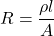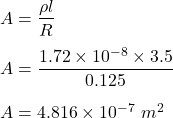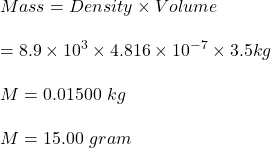## You need to produce a set of cylindrical copper wire 3.5 m long that will have a resistance of 0.125 Ω each. What will be the ma

Question

You need to produce a set of cylindrical copper wire 3.5 m long that will have a

resistance of 0.125 Ω each. What will be the mass of each of these wires?

(ρ = 1.72X10-8 Ωm, density of copper = 8.9X103 kg/m3)​

in progress 0
6 months 2021-07-27T03:35:52+00:00 1 Answers 97 views 0

1. Solution :

We know, resistance is given by :Now, we know mass of wire is given by :Hence, this is the required solution.